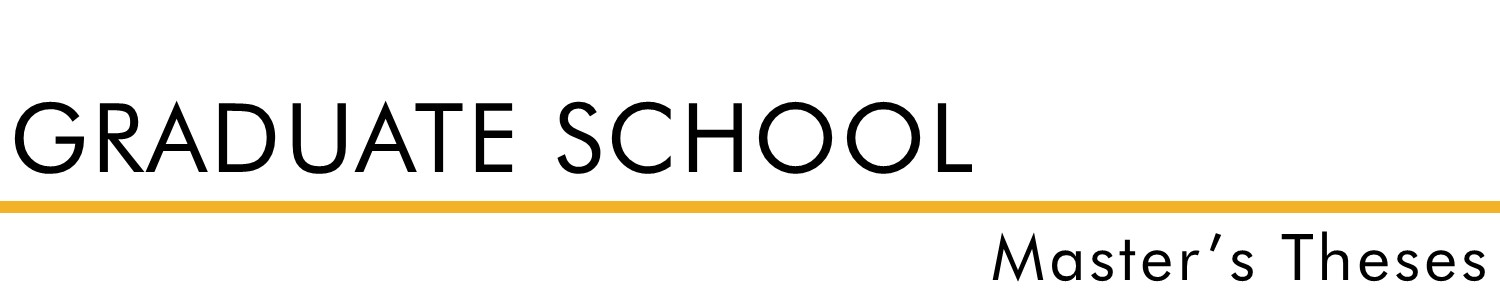#### Document Type

Thesis - campus only access

Summer 1968

#### Degree Name

Master of Science (MS)

Math

Wilmont Toalson

#### Abstract

The primary object of this study was to find n-cycles (n the number of integers in a cycle) of integers in bases three through ten using the function G(A )= [Sigma R/i = 1a 2/I; see manuscript for exact equation] with A = [Sigma R/I =1a/i (IB) R-1; see manuscript for exact equation] as the variable in each base and the ai as digits of A. N-cycles were found for every base from three to ten through the use of an IBM 1401 computer and a Fortran IV program. Since only integers in base 10 of two digits or less were used for the research, the results of the research were justified mathematically by a Convergence Theorem. This theorem shows that an integer in any base converges under successive applications of the operating function G to a number of two digits or less.

Notice: This material may be protected by copyright law (Title 17 U.S. Code).